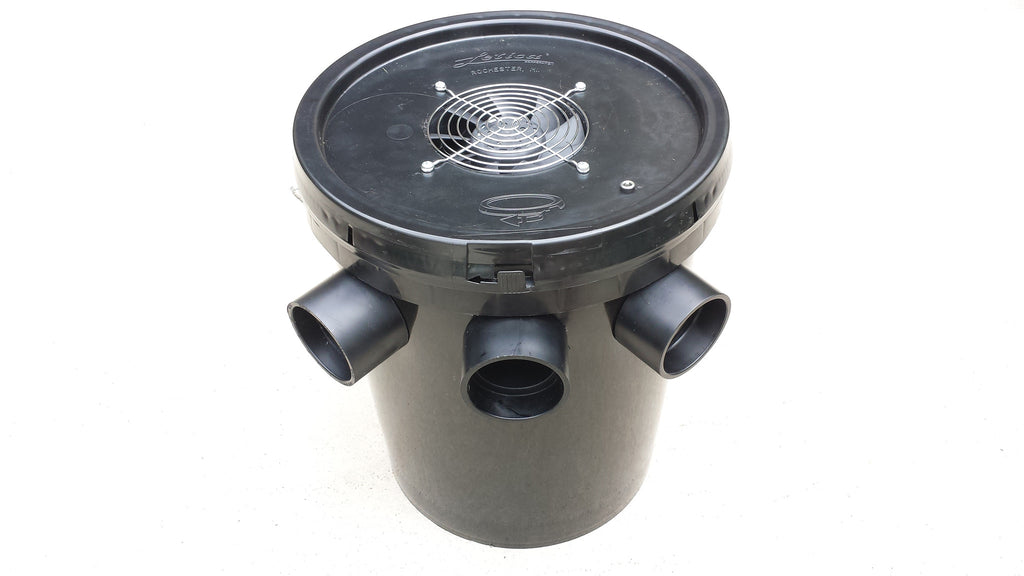# 5 Gallon Air Conditioner - Does it work? Laws of Physics

Here is the next short blog post in the 5 gallon air conditioner - does it work series.  The title of this short post is, Laws of Physics.

I have hinted at the laws of physics in the past, as these dang laws that are causing us all of our problems etc.

So, in this short post, I will go over a few of these laws that no matter how hard we try, we are just not going to overcome them.  I will try my best to keep the math to a minimum and keep it simple.

The first one is the automotive air conditioner condenser and the average automotive AC unit being rated at 1.5 tons.  There are several conversions that I will go through on this for you, to try and make it as simple as possible.

So, as discussed previously 1.5 Tons of cooling capacity equates to 18K BTU/HR.  Well, there is a conversion factor that goes from BTU/HR to HORSEPOWER.  For simplicity sake, 18K BTU/HR = 7.074 HP.

So, for simplicity, that means that it takes 7.07 HP from the engine of the car to run the air conditioning unit in the car.  An AVERAGE car makes about 150 HP, so therefore about 5% of the engine power is utilized for air conditioning.

But, there is also a conversion from BTU/HR to WATTS.  Remember watts = voltage X amperage.  So, 18K BTU/HR = approximately 5275.3 watts.  An AVERAGE accessory in and AVERAGE car, such as the cigarette lighter, is run on a 15 Amp fuse.  A 15A fuse on a 12V circuit is 180 watts.  So, it would take approximately 30 cigarette lighter plugs outlets to supply enough wattage to supply a 18K BTU/HR air conditioner for a car.

A typical car alternator the produces electricity, generally has 2 different amperage ratings.  A low speed rating and a high speed rating.  Where the low speed rating is how much amperage the alternator produces while the engine is idling, and the other is how much amperage the alternator is producing while the engine is at WOT (wide open throttle).  The AVERAGE car at the MID range (highway cruising speeds) of it's amperage output, puts out about 60 Amps.  So 60A x 12V = 720 watts.

So, that is a lot of information and numbers to digest.

So, now let's theoretically look at how I could build a 5 gallon air conditioner unit to produce 18K BTU/HR to replace your automotive air conditioner.

First off, I would need to look at the largest amperage draw I could electrically pull from the engine.  60A.  Then I could covert 720W (60Ax12V) into HP (0.96hp).  Then I could convert HP to BTU/HR = 2442.  So, going electrically,  the BIGGEST unit I could build (using the laws of physics) for an AVERAGE car with and AVERAGE electrical system is about a 2500 BTU/HR unit.  That is a little better than 1/8 the capacity of the current mechanical units.

So, electrically is the wrong approach for that application.

What about for big trucks, like Semi Trucks and delivery trucks etc.  They have alternators that mid range produce about 200 amps.  Following the same logic, about 8100 BTU/Hr would be the made AC size that could be built.  So, about 1/2 the capacity of an AVERAGE car.

So, this is just ONE of the laws of physics that we have to contend with, weight is another, efficiency is another, over time, each of these different areas will be discussed as well.